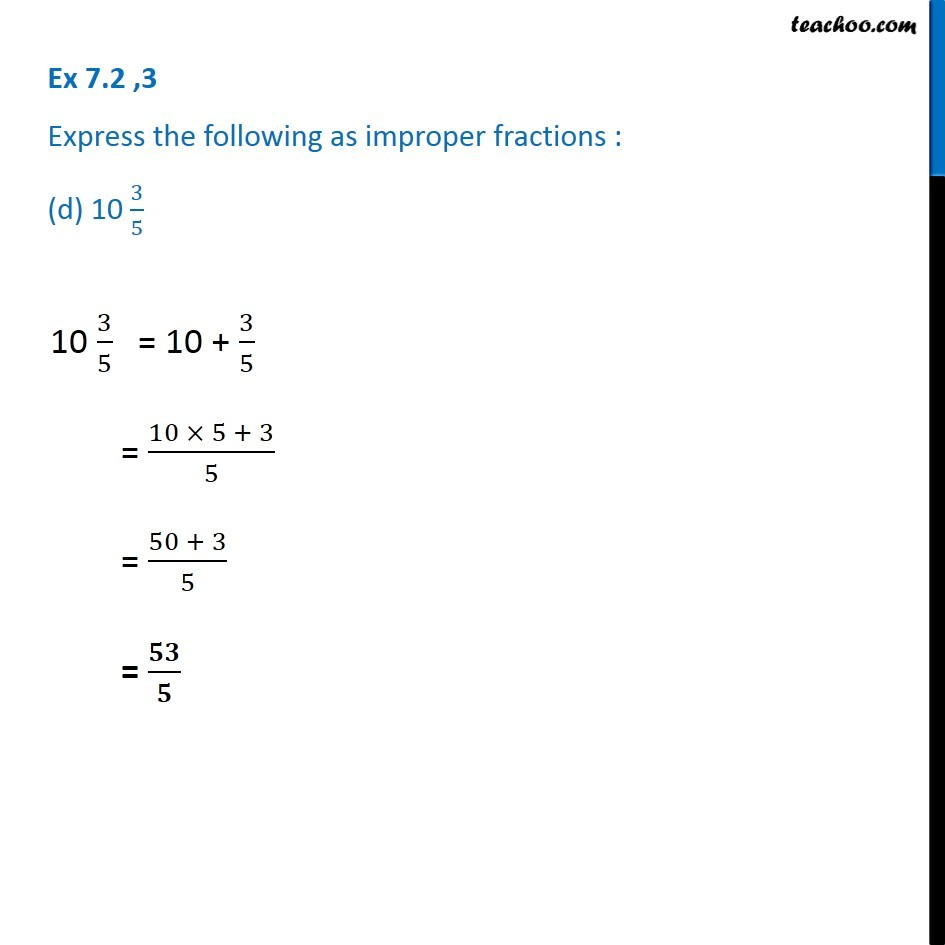Ex 7.2

Chapter 7 Class 6 Fractions
Serial order wise### Transcript

Ex 7.2 ,3 Express the following as improper fractions : (d) 10 3/5 10 3/5 = 10 + 3/5 = (10 × 5 + 3)/5 = (50 + 3)/5 = 𝟓𝟑/𝟓